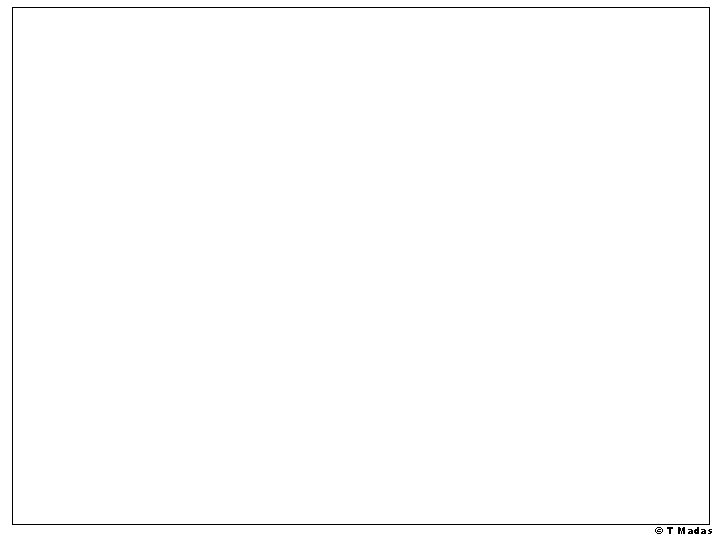# T Madas Hu Te nd nth Th red

• Slides: 40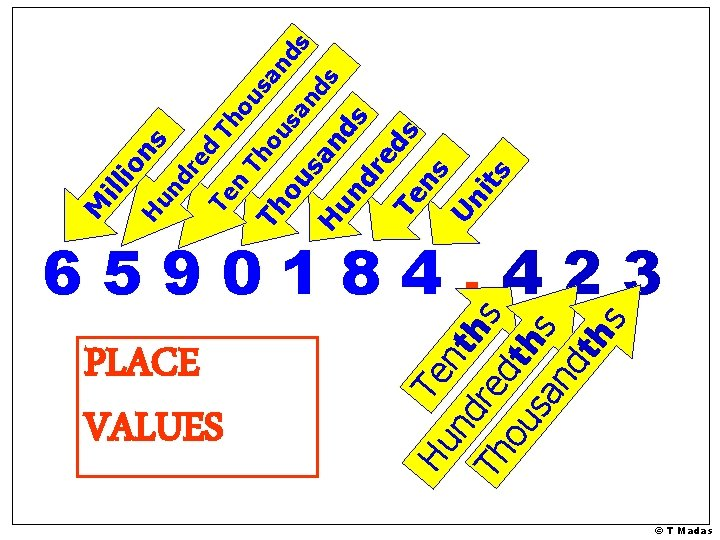Hu Te nd nth Th red s ou th sa s nd th s PLACE VALUES Th nd nd s s sa ou Th sa H un nd dr s ed Te s ns Un its ou n Te dr ed un H on s ill i M Th 6590184. 423 © T Madas9184. 723 How many hundreds in this number? 1 How many thousands in this number? 9 8 How many tenths in this number? 7 How many tens in this number? How many hundredths in this number? How many thousandths in this number? 2 3 © T Madas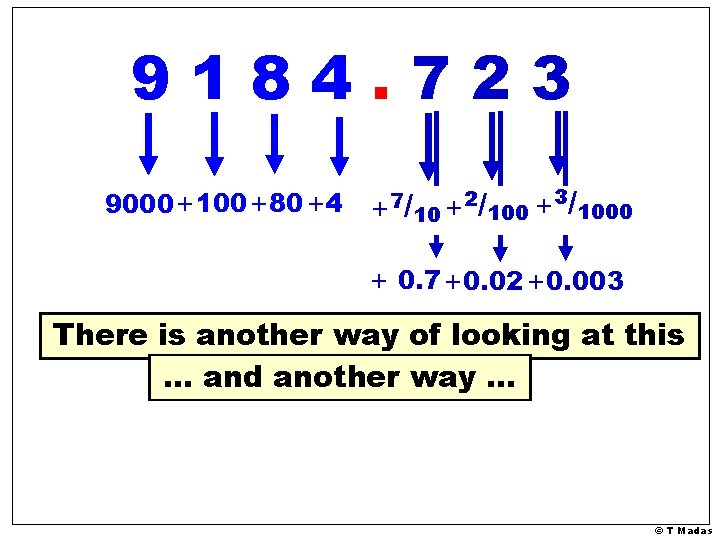9184. 723 9000+100 +80 +4 +7/10 +2/100 + /1000 3 + 0. 7 +0. 02 +0. 003 There is another way of looking at this … and another way … © T Madas9184. 723 9000+100 +80 +4 +7/10 +2/100 + /1000 3 + 0. 7 +0. 02 +0. 003 Decimals are just a special way of writing fractions out of 10, out of 1000 etc Percentages are just a special way of writing fractions out of 100. © T MadasTo multiply a whole number by: 10 We add one zero at the end of the number 100 We add two zeros at the end of the number 1000 We add three zeros at the end of the number And so on 31 x 10 = 310 514 x 100 = 51400 670 x 10 = 6700 43 x 1000 = 43000 © T MadasMultiplying two whole numbers with zeros at the end We ignore the zeros at the end of the two numbers We multiply the non-zero digits of the two numbers We put at the end of the answer zeros equal to the total zeros we ignored 20 x 30 = 600 40 x 50 = 2000 300 x 40 = 12000 20 x 120 = 2400 60 x 500 = 30000 © T MadasTo multiply any number by: 10 We “move” the decimal point one place, in the direction which makes the number bigger 100 We “move” the decimal point two places, in the direction which makes the number bigger 1000 We “move” the decimal point three places, in the direction which makes the number bigger And so on © T Madas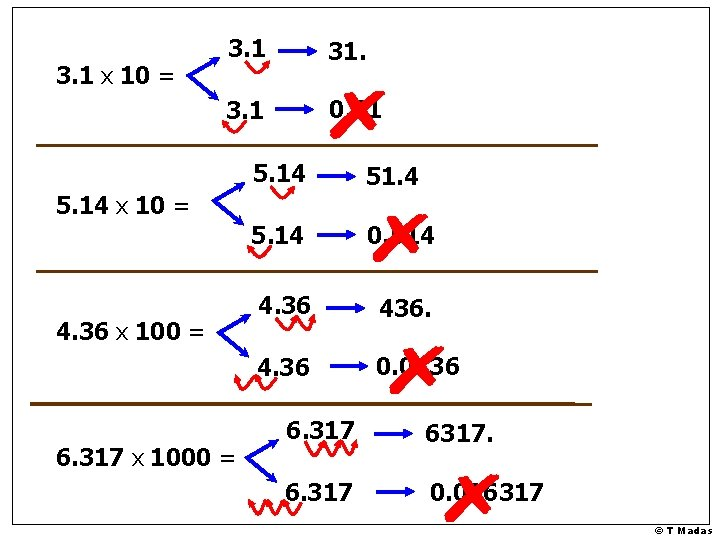3. 1 x 10 = 3. 1 31. 3. 1 0. 31 5. 14 51. 4 5. 14 0. 514 4. 36 436. 4. 36 0. 0436 5. 14 x 10 = 4. 36 x 100 = 6. 317 x 1000 = 6. 317 6317. 6. 317 0. 006317 © T Madas41 x 10 = 41. 9. 12046 x 10000 = 410. 4. 1 9. 12046 91204. 6 9. 12046 0. 000912046 And so on… © T MadasTo divide any number by: 10 We “move” the decimal point one place, in the direction which makes the number smaller 100 We “move” the decimal point two places, in the direction which makes the number smaller 1000 We “move” the decimal point three places, in the direction which makes the number smaller And so on © T Madas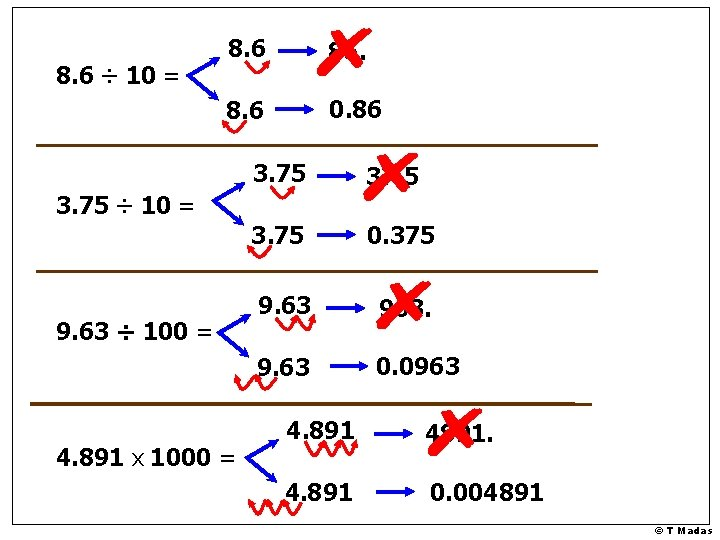8. 6 ÷ 10 = 8. 6 86. 8. 6 0. 86 3. 75 37. 5 3. 75 0. 375 9. 63 963. 9. 63 0. 0963 3. 75 ÷ 10 = 9. 63 ÷ 100 = 4. 891 x 1000 = 4. 891 4891. 4. 891 0. 004891 © T Madas314 x 10 = 3140 This is what really happens: THOUSANDS HUNDREDS TENS UNITS TENTHS 3 1 4 0 THOUSANDS HUNDREDS TENS UNITS TENTHS x 10 3 1 4 0 © T Madas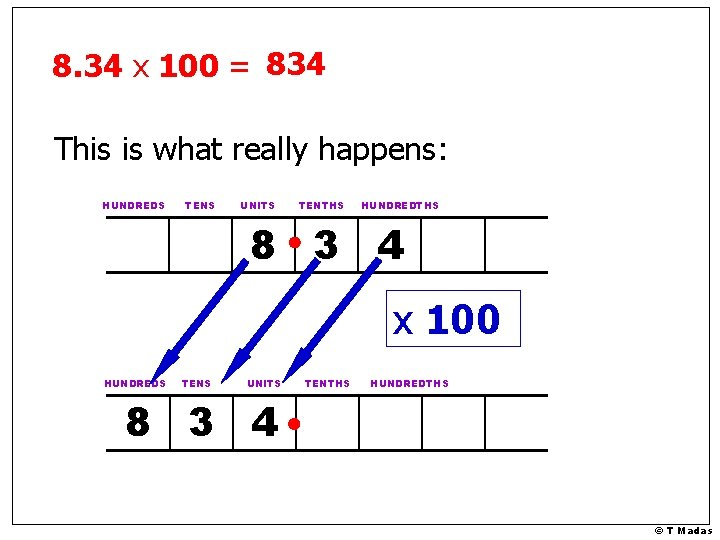8. 34 x 100 = 834 This is what really happens: HUNDREDS TENS UNITS TENTHS HUNDREDTHS 8 3 4 x 100 HUNDREDS TENS UNITS TENTHS HUNDREDTHS 8 3 4 © T Madas7. 61 x 1000 = 7610 This is what really happens: THOUSANDS HUNDREDS TENS UNITS TENTHS HUNDREDTHS 7 6 1 x 1000 THOUSANDS HUNDREDS TENS UNITS TENTHS HUNDREDTHS 7 6 1 0 © T Madas520 ÷ 10 = 52 This is what really happens: THOUSANDS HUNDREDS TENS UNITS TENTHS 5 2 0 THOUSANDS HUNDREDS TENS UNITS TENTHS ÷ 10 5 2 0 © T Madas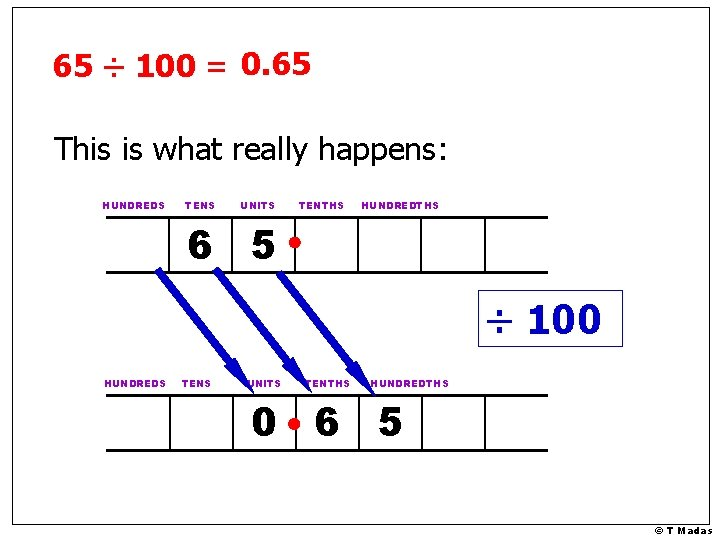65 ÷ 100 = 0. 65 This is what really happens: HUNDREDS TENS UNITS TENTHS HUNDREDTHS 6 5 ÷ 100 HUNDREDS TENS UNITS TENTHS HUNDREDTHS 0 6 5 © T MadasPractice on Multiplying & Dividing by numbers with zeros at the end © T Madas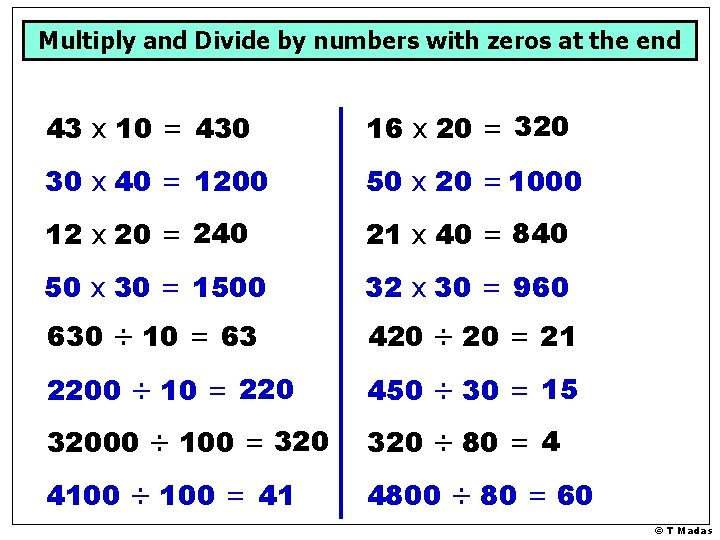Multiply and Divide by numbers with zeros at the end 43 x 10 = 430 16 x 20 = 320 30 x 40 = 1200 50 x 20 = 1000 12 x 20 = 240 21 x 40 = 840 50 x 30 = 1500 32 x 30 = 960 630 ÷ 10 = 63 420 ÷ 20 = 21 2200 ÷ 10 = 220 450 ÷ 30 = 15 32000 ÷ 100 = 320 ÷ 80 = 4 4100 ÷ 100 = 41 4800 ÷ 80 = 60 © T Madas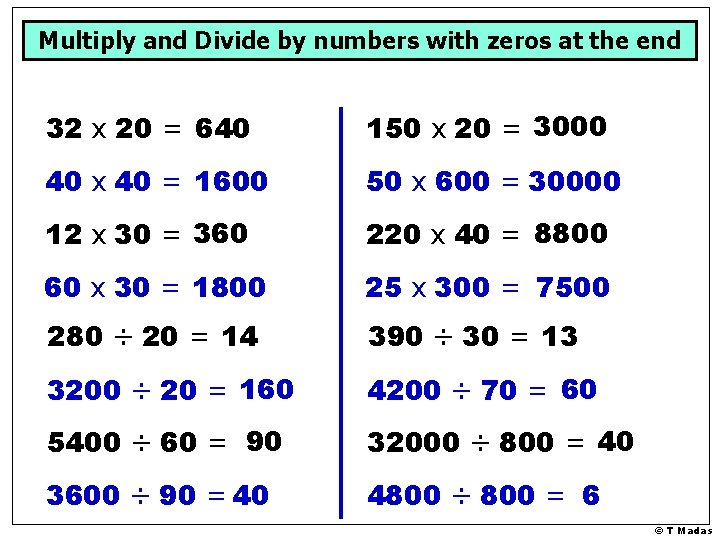Multiply and Divide by numbers with zeros at the end 32 x 20 = 640 150 x 20 = 3000 40 x 40 = 1600 50 x 600 = 30000 12 x 30 = 360 220 x 40 = 8800 60 x 30 = 1800 25 x 300 = 7500 280 ÷ 20 = 14 390 ÷ 30 = 13 3200 ÷ 20 = 160 4200 ÷ 70 = 60 5400 ÷ 60 = 90 32000 ÷ 800 = 40 3600 ÷ 90 = 40 4800 ÷ 800 = 6 © T MadasPractice on Multiplying & Dividing by numbers with zeros at the end © T MadasMultiply and Divide by numbers with zeros at the end 43 x 10 = 430 16 x 20 = 320 30 x 40 = 1200 50 x 20 = 1000 12 x 20 = 240 21 x 40 = 840 50 x 30 = 1500 32 x 30 = 960 630 ÷ 10 = 63 420 ÷ 20 = 21 2200 ÷ 10 = 220 450 ÷ 30 = 15 32000 ÷ 100 = 320 ÷ 80 = 4 4100 ÷ 100 = 41 4800 ÷ 80 = 60 © T MadasMultiply and Divide by numbers with zeros at the end 32 x 20 = 640 150 x 20 = 3000 40 x 40 = 1600 50 x 600 = 30000 12 x 30 = 360 220 x 40 = 8800 60 x 30 = 1800 25 x 300 = 7500 280 ÷ 20 = 14 390 ÷ 30 = 13 3200 ÷ 20 = 160 4200 ÷ 70 = 60 5400 ÷ 60 = 90 32000 ÷ 800 = 40 3600 ÷ 90 = 40 4800 ÷ 800 = 6 © T MadasPractice on Multiplying & Dividing by 10, 1000 … © T MadasMultiply and Divide by 10, 1000, etc 43 x 10 = 430 8. 6 x 10 = 86 17 x 100 = 1700 4. 7 x 100 = 470 123 x 10 = 1230 1. 25 x 10 =12. 5 58 x 1000 = 58000 2. 813 x 1000 = 2813 570 ÷ 10 = 57 42 ÷ 10 = 4. 2 8600 ÷ 10 = 860 465 ÷ 100 = 4. 65 32000 ÷ 100 = 320 ÷ 100 = 3. 2 4100 ÷ 100 = 41 4109 ÷ 100 = 41. 09 © T Madas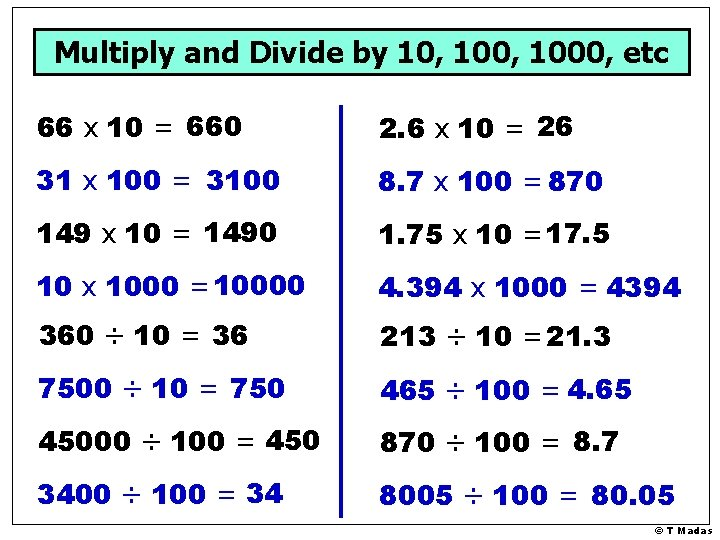Multiply and Divide by 10, 1000, etc 66 x 10 = 660 2. 6 x 10 = 26 31 x 100 = 3100 8. 7 x 100 = 870 149 x 10 = 1490 1. 75 x 10 =17. 5 10 x 1000 = 10000 4. 394 x 1000 = 4394 360 ÷ 10 = 36 213 ÷ 10 = 21. 3 7500 ÷ 10 = 750 465 ÷ 100 = 4. 65 45000 ÷ 100 = 450 870 ÷ 100 = 8. 7 3400 ÷ 100 = 34 8005 ÷ 100 = 80. 05 © T MadasMultiply and Divide by 10, 1000, etc 131 x 10 = 1310 9. 63 x 10 = 96. 3 30 x 40 = 1200 5. 52 x 100 = 552 20 x 50 = 1000 4. 05 x 10 =40. 5 70 x 500 = 35000 9. 506 x 1000 = 9506 930 ÷ 10 = 93 7. 002 x 100 = 700. 2 1800 ÷ 10 = 180 35 ÷ 10 = 3. 5 2. 7 x 10 = 27 809 ÷ 100 = 8. 09 4. 1 x 10 = 41 1876 ÷ 1000 = 1. 876 © T MadasMultiply and Divide by 10, 1000, etc 16 x 10 = 160 8. 65 x 10 = 86. 5 123 x 10 = 1230 2. 71 x 100 = 271 48 x 100 = 4800 2. 25 x 10 =22. 5 5. 8 x 10 = 58 4. 916 x 1000 = 4916 570 ÷ 10 = 57 52 ÷ 100 = 0. 52 8600 ÷ 10 = 860 375 ÷ 100 = 3. 75 32 ÷ 10 = 3. 2 665 ÷ 100 = 6. 65 41 ÷ 10 = 4. 1 792 ÷ 1000 = 0. 792 © T Madas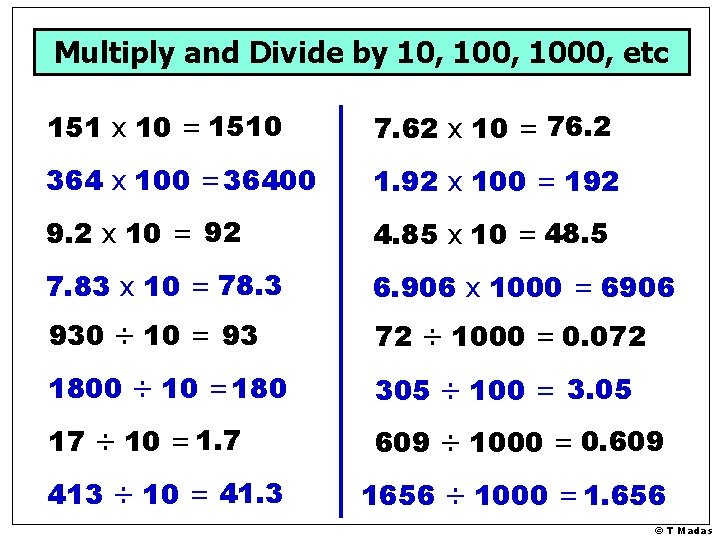Multiply and Divide by 10, 1000, etc 151 x 10 = 1510 7. 62 x 10 = 76. 2 364 x 100 = 36400 1. 92 x 100 = 192 9. 2 x 10 = 92 4. 85 x 10 = 48. 5 7. 83 x 10 = 78. 3 6. 906 x 1000 = 6906 930 ÷ 10 = 93 72 ÷ 1000 = 0. 072 1800 ÷ 10 = 180 305 ÷ 100 = 3. 05 17 ÷ 10 = 1. 7 609 ÷ 1000 = 0. 609 413 ÷ 10 = 41. 3 1656 ÷ 1000 = 1. 656 © T Madas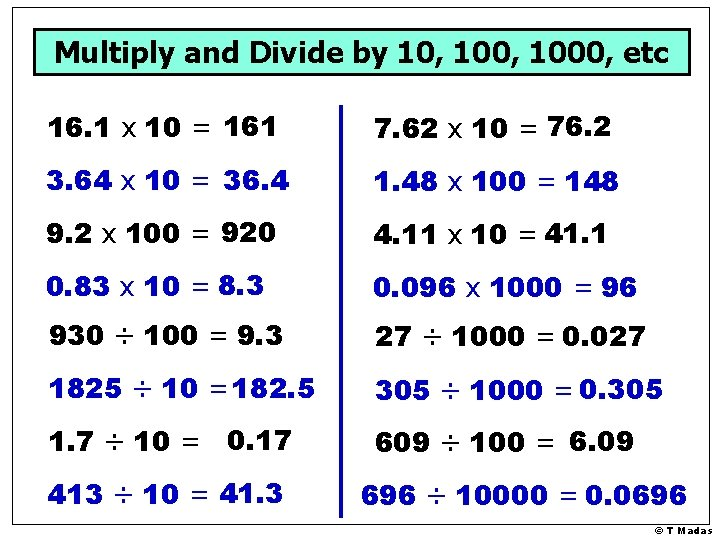Multiply and Divide by 10, 1000, etc 16. 1 x 10 = 161 7. 62 x 10 = 76. 2 3. 64 x 10 = 36. 4 1. 48 x 100 = 148 9. 2 x 100 = 920 4. 11 x 10 = 41. 1 0. 83 x 10 = 8. 3 0. 096 x 1000 = 96 930 ÷ 100 = 9. 3 27 ÷ 1000 = 0. 027 1825 ÷ 10 = 182. 5 305 ÷ 1000 = 0. 305 1. 7 ÷ 10 = 0. 17 609 ÷ 100 = 6. 09 413 ÷ 10 = 41. 3 696 ÷ 10000 = 0. 0696 © T Madas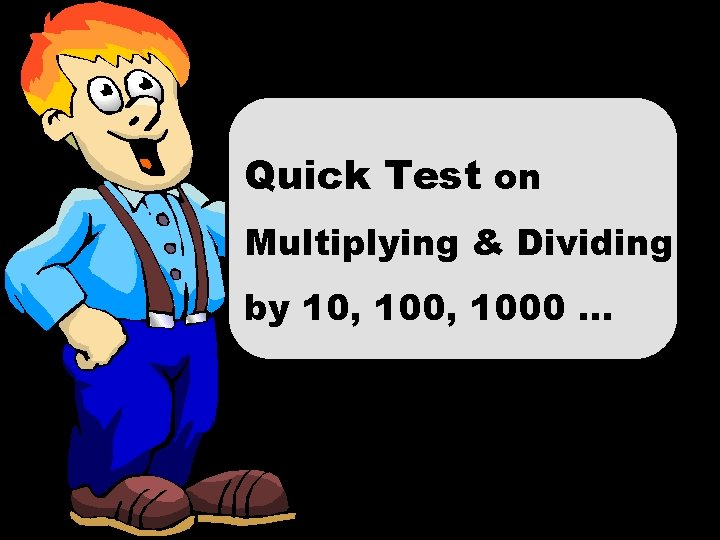Quick Test on Multiplying & Dividing by 10, 1000 … © T MadasMultiply and Divide by 10, 1000, etc 43 x 10 = 430 8. 6 x 10 = 86 17 x 100 = 1700 4. 7 x 100 = 470 123 x 10 = 1230 1. 25 x 10 =12. 5 58 x 1000 = 58000 2. 813 x 1000 = 2813 570 ÷ 10 = 57 42 ÷ 10 = 4. 2 8600 ÷ 10 = 860 465 ÷ 100 = 4. 65 32000 ÷ 100 = 320 ÷ 100 = 3. 2 4100 ÷ 100 = 41 4109 ÷ 100 = 41. 09 © T MadasMultiply and Divide by 10, 1000, etc 66 x 10 = 660 2. 6 x 10 = 26 31 x 100 = 3100 8. 7 x 100 = 870 149 x 10 = 1490 1. 75 x 10 =17. 5 10 x 1000 = 10000 4. 394 x 1000 = 4394 360 ÷ 10 = 36 213 ÷ 10 = 21. 3 7500 ÷ 10 = 750 465 ÷ 100 = 4. 65 45000 ÷ 100 = 450 870 ÷ 100 = 8. 7 3400 ÷ 100 = 34 8005 ÷ 100 = 80. 05 © T Madas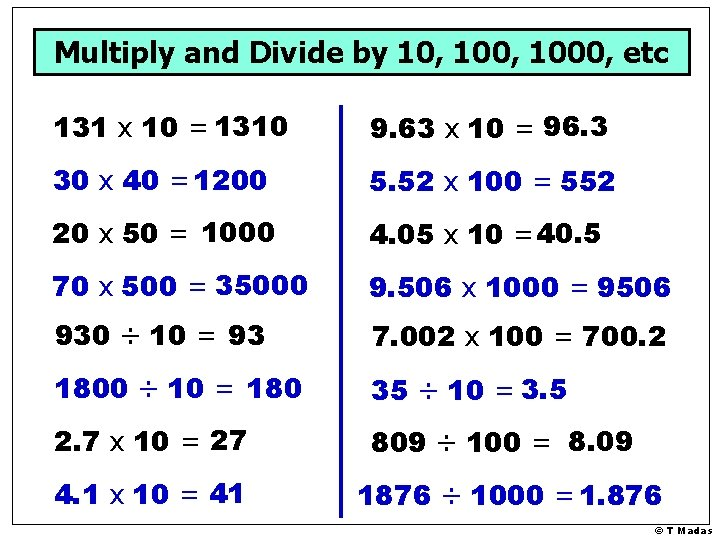Multiply and Divide by 10, 1000, etc 131 x 10 = 1310 9. 63 x 10 = 96. 3 30 x 40 = 1200 5. 52 x 100 = 552 20 x 50 = 1000 4. 05 x 10 =40. 5 70 x 500 = 35000 9. 506 x 1000 = 9506 930 ÷ 10 = 93 7. 002 x 100 = 700. 2 1800 ÷ 10 = 180 35 ÷ 10 = 3. 5 2. 7 x 10 = 27 809 ÷ 100 = 8. 09 4. 1 x 10 = 41 1876 ÷ 1000 = 1. 876 © T MadasMultiply and Divide by 10, 1000, etc 16 x 10 = 160 8. 65 x 10 = 86. 5 123 x 10 = 1230 2. 71 x 100 = 271 48 x 100 = 4800 2. 25 x 10 =22. 5 5. 8 x 10 = 58 4. 916 x 1000 = 4916 570 ÷ 10 = 57 52 ÷ 100 = 0. 52 8600 ÷ 10 = 860 375 ÷ 100 = 3. 75 32 ÷ 10 = 3. 2 665 ÷ 100 = 6. 65 41 ÷ 10 = 4. 1 792 ÷ 1000 = 0. 792 © T MadasMultiply and Divide by 10, 1000, etc 151 x 10 = 1510 7. 62 x 10 = 76. 2 364 x 100 = 36400 1. 92 x 100 = 192 9. 2 x 10 = 92 4. 85 x 10 = 48. 5 7. 83 x 10 = 78. 3 6. 906 x 1000 = 6906 930 ÷ 10 = 93 72 ÷ 1000 = 0. 072 1800 ÷ 10 = 180 305 ÷ 100 = 3. 05 17 ÷ 10 = 1. 7 609 ÷ 1000 = 0. 609 413 ÷ 10 = 41. 3 1656 ÷ 1000 = 1. 656 © T MadasMultiply and Divide by 10, 1000, etc 16. 1 x 10 = 161 7. 62 x 10 = 76. 2 3. 64 x 10 = 36. 4 1. 48 x 100 = 148 9. 2 x 100 = 920 4. 11 x 10 = 41. 1 0. 83 x 10 = 8. 3 0. 096 x 1000 = 96 930 ÷ 100 = 9. 3 27 ÷ 1000 = 0. 027 1825 ÷ 10 = 182. 5 305 ÷ 1000 = 0. 305 1. 7 ÷ 10 = 0. 17 609 ÷ 100 = 6. 09 413 ÷ 10 = 41. 3 696 ÷ 10000 = 0. 0696 © T Madas